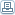# C++ Function Templates

 By: MuthukumarC++ Function templates are those functions which can handle different data types without separate code for each of them. For a similar operation on several kinds of data types, a programmer need not write different versions by overloading a function. It is enough if he writes a C++ template based function. This will take care of all the data types.There are two types of templates in C++, viz., function templates and class templates. This article deals with only the function templates.There are lot of occasions, where we might need to write the same functions for different data types. A favorite example can be addition of two variables. The variable can be integer, float or double. The requirement will be to return the corresponding return type based on the input type. If we start writing one function for each of the data type, then we will end up with 4 to 5 different functions, which can be a night mare for maintenance. C++ templates come to our rescue in such situations. When we use C++ function templates, only one function signature needs to be created. The C++ compiler will automatically generate the required functions for handling the individual data types. This is how a programmer's life is made a lot easier.C++ Template functions - Details: Let us assume a small example for Add function. If the requirement is to use this Add function for both integer and float, then two functions are to be created for each of the data type (overloading).` int Add(int a,int b) { return a+b;} // function Without C++ template float Add(float a, float b) { return a+b;} // function Without C++ template`If there are some more data types to be handled, more functions should be added. But if we use a c++ function template, the whole process is reduced to a single c++ function template. The following will be the code fragment for Add function.` template T Add(T a, T b) //C++ function template sample { return a+b; }`This c++ function template definition will be enough. Now when the integer version of the function, the compiler generates an Add function compatible for integer data type and if float is called it generates float type and so on.Here T is the typename. This is dynamically determined by the compiler according to the parameter passed. The keyword class means, the parameter can be of any type. It can even be a class. C++ Template functions - Applicability:C++ function templates can be used wherever the same functionality has to be performed with a number of data types. Though very useful, lots of care should be taken to test the C++ template functions during development. A well written c++ template will go a long way in saving time for programmers.
Computers and The Internet